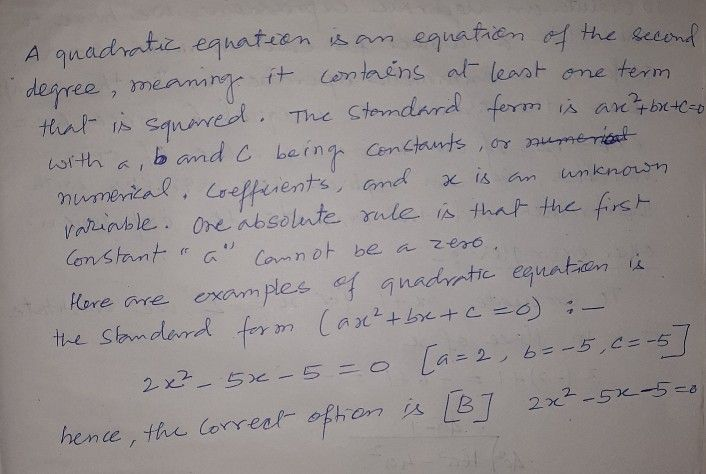Symbol
Problem$ions$ $c$ Read each item carefully. Shade the letter that corresponds to your choice on your answer $shcc1$ Which of the $fo1losy^{ing}$ is an example $0a$ quadratic equation? A. $2x^{2}$ $-x>3$ $B$ $2x^{2}$ - $52$ $-$ - $5$ $=$ $0$ $C$ $5x-5=0$ $D$ $2x^{4}+1=0$ $21$ Which of the $fo1losyina$ quadratic eouations
7th-9th grade
Other
Search count: 105
SolutionQanda teacher - Anisha